Science, Maths & Technology

### Become an OU student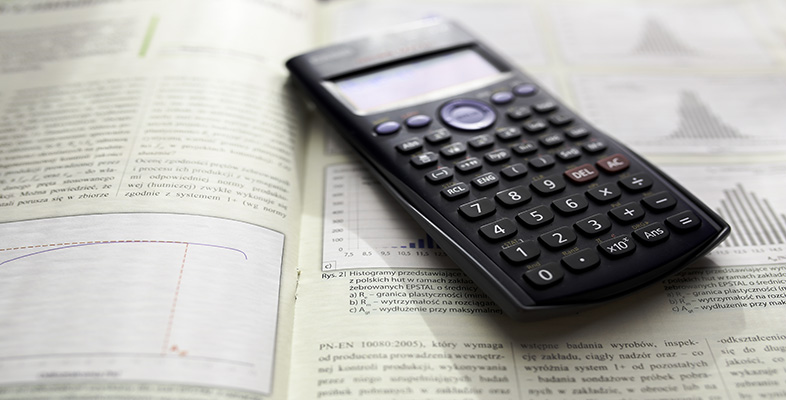Using a scientific calculator

Start this free course now. Just create an account and sign in. Enrol and complete the course for a free statement of participation or digital badge if available.

# 5.1 Inputting numbers in scientific notation to your calculator

Numbers expressed in scientific notation can be input directly to the calculator by using the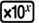key on the bottom row of keys. For example,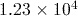can be entered using the key sequence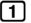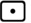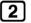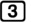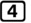.

## Activity 13 Calculating with scientific notation

Use the scientific notation functions of your calculator to calculate each of the following, giving your answer in both scientific and ordinary forms.

1.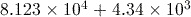2.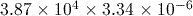3.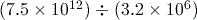1.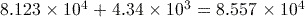or 85570
2.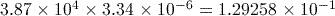or 0.129258
3.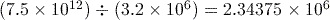or 2343750